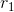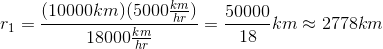# AP Physics C: Mechanics : Using Angular Momentum Equations

## Example Questions

### Example Question #71 : Ap Physics C

Two solid cylinderical disks have equal radii. The first disk is spinning clockwise at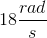and the second disk is spinning counterclockwise at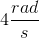. The second disk has a mass three times larger than the first. If both spinning disks are combined to form one disk, they end up rotating at the same angular velocity and same direction. Find this angular velocity after combination.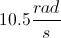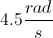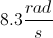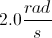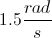Explanation:

For the first disk, we have the information below.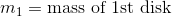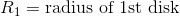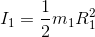For the second disk, we have the information below.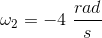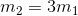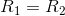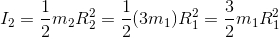To do this problem, we use conservation of angular momentum. Before the disks are put in contact, the initial total angular momentum is given by the equation below.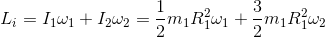It is just the sum of the angular momentums of each disk. When the disks are combined together, then final angular momentum can be found by the following equation.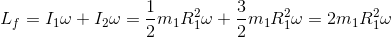Set this equal to the initial angular momentum.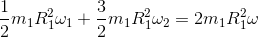Simplify and solve for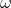.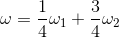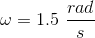### Example Question #2 : Using Angular Momentum Equations

A piece of space debris is travelling in an elliptical orbit around a planet. At its closest point to the planet, the debris is travelling at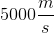. When the debris is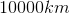from the planet, it travels at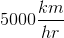. How close does the debris get to the planet in its orbit?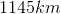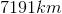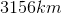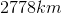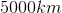Explanation:

The equation for conservation of angular momentum is: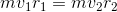This means that the angular momentum of the object at the two points in its orbit must be the same. Since the mass of the debris does not change, this gives us an equality of the product of the velocity and distance of the debris at any two points of its orbit: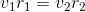Plug in known values.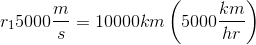There is disagreement between units; the velocities are given in both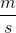and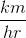. Glance down at the answer choices and note that they are all lengths in kilometers.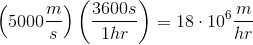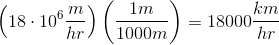Solve for